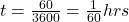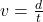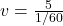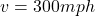A cheetah runs 5 miles in 60 seconds. How fast did the cheetah run in miles per hour? Convert seconds in to hours first then solve.

Question

A cheetah runs 5 miles in 60 seconds. How fast did the cheetah run in miles per hour? Convert seconds in to hours first then solve.

in progress 0
5 months 2021-08-09T15:47:51+00:00 1 Answers 4 views 0

Speed of the cheetah is 300 miles per hour

Explanation:

As we know that the speed is defined as the ratio of distance and time

here we know that Cheetah runs a total distance of d = 5 miles

also the time taken by the cheetah is

t = 60 s

now we haveso here we have